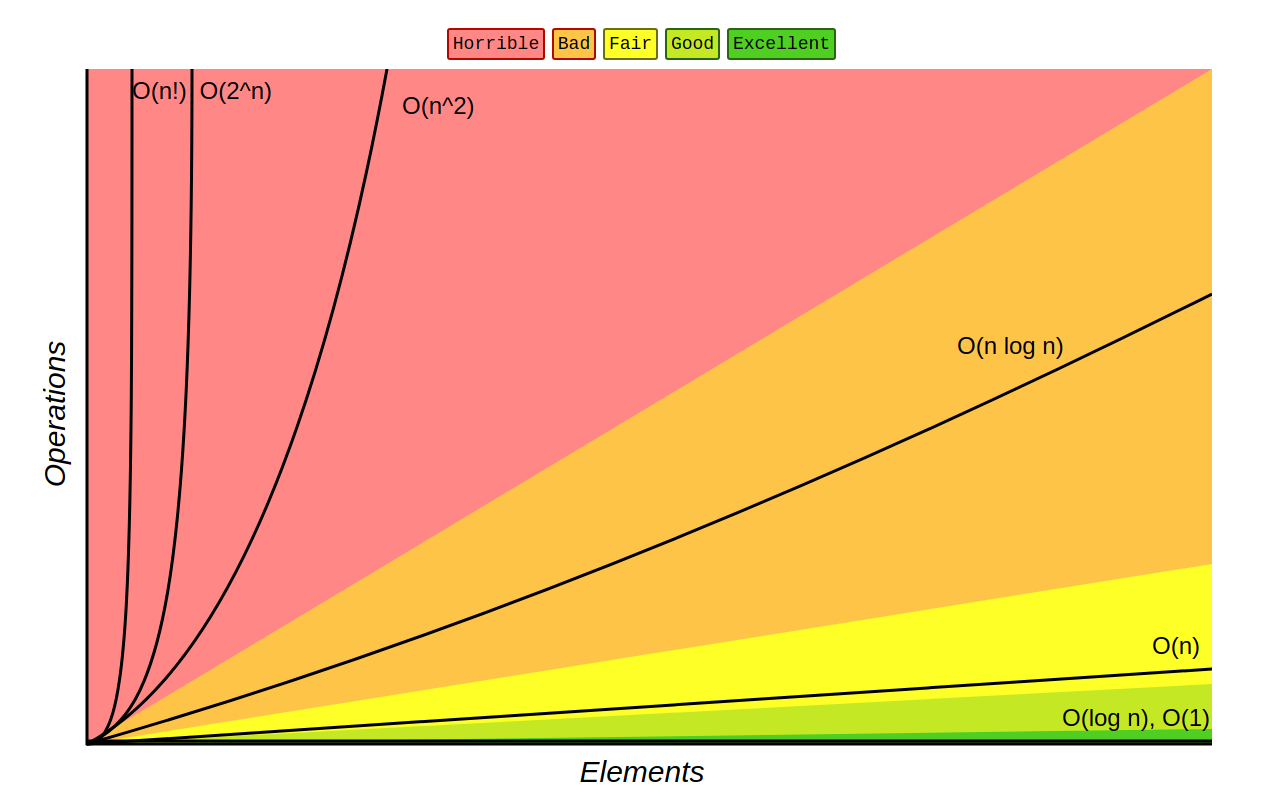As a web developer, I very rarely find myself deeply analyzing algorithms. However, a basic understanding of Big-O analysis can be really useful for software engineers. It provides a common language for us to discuss the performance of our code and the potential ways we can improve it.

## What is Big-O notation?

An algorithm is a set of operations describing how to solve a problem. Generally, the more complex an algorithm, the longer it takes to run. How do we describe an algorithm’s complexity?

Big-O notation is a way to express an algorithm’s complexity in terms of the size of its input. It gives us an idea of how an algorithm scales as its input grows. An algorithm can be classified into one of several Big-O functions, usually written as `O(n)` or `O(n2)`. With these functions, we can compare the complexity (and performance) of our algorithms.

## A Basic Example

Here’s a simple search function:

Let’s walk through the steps for determining this function’s Big-O complexity:

### Determine the Input Size

This one is relatively simple: the number of elements in `items` is our input size, `n`.

### Only Consider the Worst-Case Scenario

If the first item in `items` is equal to `target`, the function returns in only one operation. This is the best-case scenario. We only care about the worst-case scenario, which occurs when `target` doesn’t exist in `items` at all. In this situation, we end up iterating through the entire list.

### Count the Number of Operations

We can express the number of operations performed as a function of `n`. We know our worst-case scenario involves iterating the entire list. So, the Big-O complexity of `linear_search` is `O(n)`. In other words, our algorithm completes in “linear time”. If `items` has 10 items, we do 10 operations. If it has 1000 items, we do 1000 operations. And so on.

### Ignore Insignificant Terms

Big-O notation is an approximation on large values of `n`. This means as `n` gets extremely large, some terms end up being less significant than others. So, we just drop them. Also, we are evaluating operations without any specific unit of “cost”. This means coefficients aren’t very meaningful. We can drop them as well.

For example:

`O(23n2 + 45n + 100000)` is reduced to `O(n2)`

## Common Big-O Classes

With a basic understanding of Big-O analysis, let’s look at some common classes of complexities:

### O(1)

This is known as “constant time” because the algorithm completes in the same amount of time no matter what the size of the input is. An example of this is pushing or popping from a stack:

Regardless of how big `items` is, we still yield the same amount of operations.

### O(log(n))

Let’s revisit our searching algorithm from before. What if we assumed our input is sorted? With this assumption, we can reduce the runtime complexity of our search by taking a different approach:

This approach is commonly known as “divide and conquer”. Rather than looping through all items, divide-and-conquer algorithms halve the input size (often recursively) at each step.

This results in an algorithmic complexity resembling a logarithmic function, thus the Big-O complexity of `O(log(n))`.

### O(nlog(n))

Some algorithms involve some kind of divide-and-conquer strategy combined with linear processing of all elements. This is very common in sorting algorithms. An example of this is `merge_sort`:

In `merge_sort`, we implement our divide-and-conquer strategy. As we’ve learned, this has a runtime complexity of `O(log(n))`.

The “merging” occurs in `merge`. In this function, we compare the heads of each array, popping the smaller element, and pushing it into a new array. The result is a merged, sorted array. Since we process each element in both arrays, this function has a runtime complexity of `O(n)`.

When all is said in done, we get an overall runtime complexity of `O(nlog(n))`.

### O(nx)

A big clue to identifying algorithms with `O(nx)` complexity is nested loops. We usually say these algorithms complete in “polynomial time”. Inefficient sorting algorithms often fall into this class:

In `bubble_sort`, for each item in `items`, we make another iteration back over `items` and swap elements according to their value. This “bubbles up” each item to its correct position in the array. Since there is a loop within a loop, this function yields total of `n * n` operations. This means the Big-O complexity of `bubble_sort` is `O(n * n)`, or `O(n2)`.

## Visualizing Big-O

We can easily compare different Big-O complexities by graphing them:(Source: http://bigocheatsheet.com/)

If it can be helped, we should strive to write functions with complexities `O(nlog(n))` or better. These classes of algorithms scale much better than their polynomial and exponential counterparts.

## Conclusion

Obviously, this is a very basic explanation for Big-O notation. There’s much more math involved in describing and proving these concepts. Here are some great resources for learning more about Big-O notation and analysis: